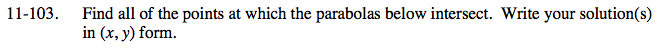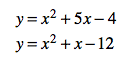Home > AC > Chapter 11 > Lesson 11.3.1 > Problem11-103

11-103.

Find all of the points at which the parabolas below intersect. Write your solution(s) in (x, y) form. 11-103 HW eTool (Desmos). Homework Help ✎

y = x2 + 5x − 4
y = x2 + x − 12Set the equations equal to each other using the Equal Values Method.

Subtract x2 from both sides.

Subtract x from both sides.

Add 4 to both sides.

Divide by 4 on both sides.

Substitute the x-value you found into one of the original equations.

x2 + x − 12 = x2 + 5x − 4

x − 12 = 5x − 4

−12 = 4x − 4

−8 = 4x

−2 = x

y = (−2)2 + 5(−2) − 4
y = 4 − 10 − 4
y = −10

They intersect at (−2, −10)